# 机器学习【九】数据表达与特征工程

• 如何将不同的特征进行转换
• 如何合理表达数据
• 如何进行特征选择

1.使用哑变量转换类型特征

#导入pandas
import pandas as pd
#手工输入一个数据表
fruits = pd.DataFrame({'数值特征':[5,6,7,8,9],'类型特征':['西瓜','香蕉','橘子','苹果','葡萄']})
#显示fruits数据表
display(fruits)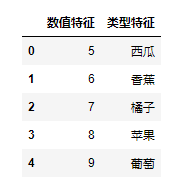【结果分析】

上图就是我们使用pandas的DataFrame生成一个完整数据集，其中包括整型数值特征[5,6,7,8],还包括字符串组成的类型特征

#转化数据表中的字符串为数值
fruits_dum = pd.get_dummies(fruits)
#显示转化后的数据表
display(fruits_dum)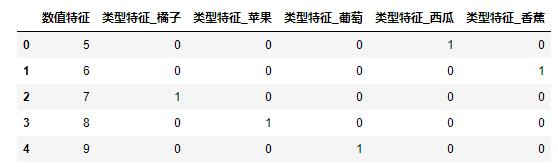【结果分析】

已全部变成 0 1 的数值变量，或者说是一个 稀疏矩阵

假设希望把数值特征也进行get_dummies转换怎么办？

#令程序将数值也看作字符串
fruits['数值特征'] = fruits['数值特征'].astype(str)
#在用get_dummies转化字符串
pd.get_dummies(fruits,columns=['数值特征'])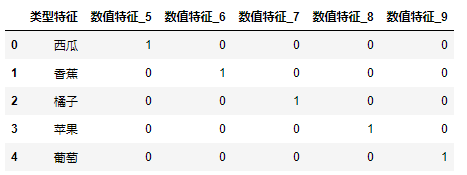【结果分析】

首先使用 .astype指定了“数值特征”这一列是字符串类型的数据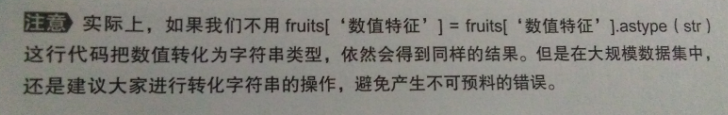2.对数据进行装箱处理

#导入numpy
import numpy as np
#导入画图工具
import matplotlib.pyplot as plt
#生成随机数列
rnd = np.random.RandomState(38)
x= rnd.uniform(-5,5,size=50)
#向数据集中添加噪声
y_no_noise = (np.cos(6*x)+x)
X = x.reshape(-1,1)
y = (y_no_noise + rnd.normal(size=len(x))/2)
#绘制图形
plt.plot(X,y,'o',c='r')
plt.show()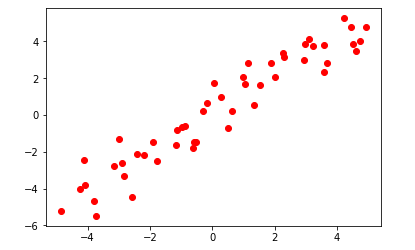【此段代码用来生成随机数据】

#导入神经网络
from sklearn.neural_network import MLPRegressor
#导入KNN
from sklearn.neighbors import KNeighborsRegressor
#生成一个等差数列
line = np.linspace(-5,5,1000,endpoint=False).reshape(-1,1)
#分别用两种算法拟合数据
mlpr = MLPRegressor().fit(X,y)
knr = KNeighborsRegressor().fit(X,y)
#绘制图形
plt.plot(line,mlpr.predict(line),label='MLP')
plt.plot(line,knr.predict(line),label='KNN')
plt.plot(X,y,'o',c='r')
plt.legend(loc='best')
plt.show()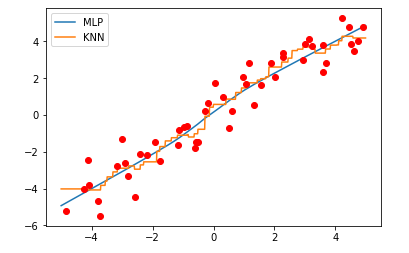【结果分析】

MLP产生的回归线非常接近线性模型的结果

KNN相对复杂点，视图覆盖更多的数据点

#设置箱体数为11
bins = np.linspace(-5,5,11)
#将数据进行装箱操作
target_bin = np.digitize(X,bins=bins)
#打印装箱数据范围
print('装箱数据范围:\n',bins)
#打印前十个数据的特征值
print('前十个数据的特征值:\n',X[:10])
print('-'*20)
#找到所在的箱子
print('找到所在的箱子：\n',target_bin[:10])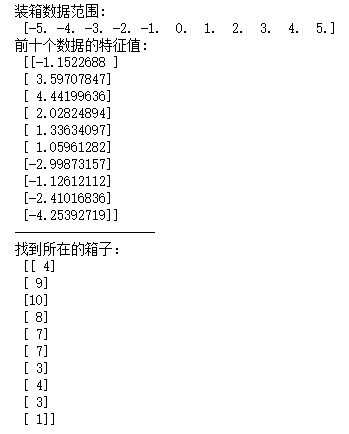生成这个实验数据集的时候，是在-5~5之间随机生成了50个数据点，因此在生成箱子的时候，也指定范围为-5~5之间，生成11个元素的等差数列，这样每两个数值之间就形成了一个箱子，共10个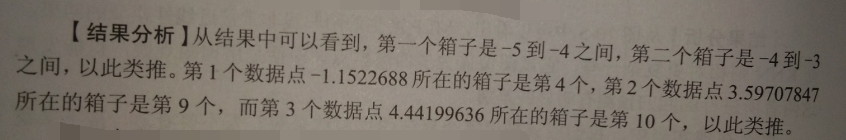OneHotEncoder和pandas的get_dummies功能差不多，但是OneHotEncoder目前只能用于整型数值的类型变量

#导入独热编码
from sklearn.preprocessing import OneHotEncoder
onehot = OneHotEncoder(sparse = False)
onehot.fit(target_bin)
#使用独热编码转换数据
X_in_bin = onehot.transform(target_bin)
#打印结果
#装箱后的数据形态
print('装箱后的数据形态:\n',X_in_bin.shape)
#装箱后的前十个数据点
print('装箱后的前十个数据点:\n',X_in_bin[:10])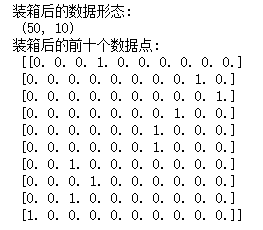【结果分析】

虽然数据集中样本的数量仍然是50个，但特征数变成了10个【箱子10个】

这样一来，相当于把原先数据集中的连续特征转化成了类别特征

#使用独热编码进行数据表达
new_line = onehot.transform(np.digitize(line,bins=bins))
#使用新的数据来训练模型
new_mlpr = MLPRegressor().fit(X_in_bin,y)
new_knr = KNeighborsRegressor().fit(X_in_bin,y)
#绘制图形
plt.plot(line,new_mlpr.predict(new_line),label='New MLP')
plt.plot(line,new_knr.predict(new_line),label='New KNN')
plt.plot(X,y,'o',c='r')
#设置图形
plt.legend(loc='best')
plt.show()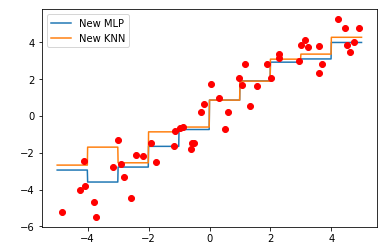【结果分析】

MLP和KNN模型更相似，当x>0时，两模型几乎重合

• 纠正模型过拟合和欠拟合问题
• 尤其针对大规模高纬度的数据集使用线性模型的时候，可以大幅度提高预测的准确率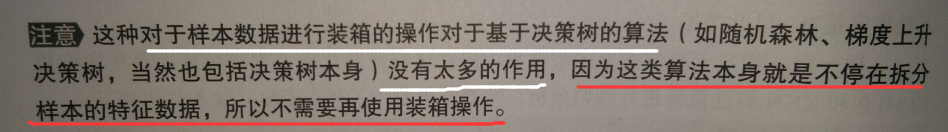数据“升维”

1.向数据集添加交互式特征

【通过np.hstack将两个数组堆叠一起】
#手工生成两个数组
array_1 = [1,2,3,4,5]
array_2 = [6,7,8,9,0]
#使用hstack 将两个数组进行堆叠
array_3 = np.hstack((array_1,array_2))
#打印结果
print('将数组2添加到数据1中得到:',array_3)
将数组2添加到数据1中得到: [1 2 3 4 5 6 7 8 9 0]
【结果分析】

#将原始数据和装箱后的数据进行堆叠
#将原始数据和装箱后的数据进行堆叠
X_stack = np.hstack([X,X_in_bin])
print(X_stack.shape)
(50, 11)
【结果分析】

X_stack 的数量仍然是50个，而特征数量11个

用X_stack训练模型：
#将数据进行堆叠
line_stack = np.hstack([line,new_line])
#重新训练模型
mlpr_interact = MLPRegressor().fit(X_stack,y)
#绘制图形
plt.plot(line,mlpr_interact.predict(line_stack),label='MLP for interaction')
plt.ylim(-4,4)
for vline in bins:
plt.plot([vline,vline],[-5,5],':',c='k')
plt.legend(loc='lower right')
plt.plot(X,y,'o',c='r')
plt.show()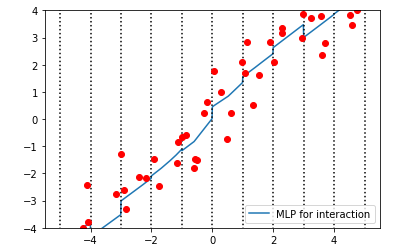【结果分析】

对比本节开始的MLP模型，我们发现在每个数据的箱体中，前者的模型是水平的，此图中是倾斜的——>  在添加交互式特征后，在每个数据所在的箱体中，MLP模型增加了斜率，复杂度有所提高

#使用新的堆叠方式处理数据
X_multi = np.hstack([X_in_bin,X*X_in_bin])
#打印结果
print(X_multi.shape)
print(X_multi)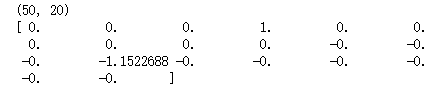【结果分析】

#重新训练模型
mlpr_multi = MLPRegressor().fit(X_multi,y)
line_multi = np.hstack([new_line,line * new_line])
#绘制图形
plt.plot(line,mlpr_multi.predict(line_multi),label='MLP Regressor')
for vline in bins:
plt.plot([vline,vline],[-5,5],':',c='gray')
plt.plot(X,y,'o',c='r')
plt.legend(loc='lower right')
plt.show()【结果分析】

这样处理的目的：

2.向数据集添加多项式特征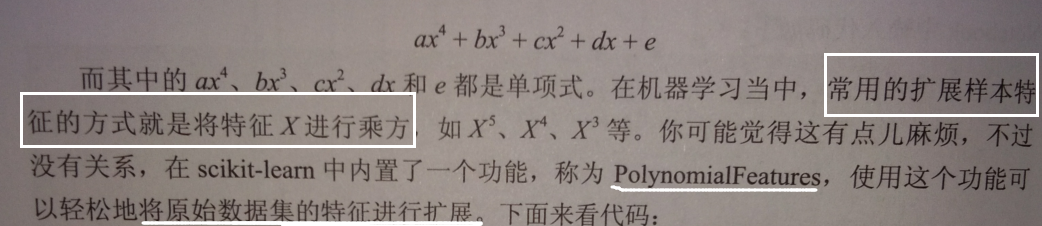#导入多项式特征工具
from sklearn.preprocessing import PolynomialFeatures
#向多项式添加多项式特征
poly = PolynomialFeatures(degree=20,include_bias=False)
X_poly = poly.fit_transform(X)
print(X_poly.shape)
(50, 20)

【结果分析】

degree参数 = 20 ,生成20个特征
include_bias = False ，如果设定为True时，PolynomialFeatures只会为数据集添加数值为1的特征

PolynomialFeatures对数据进行怎样的调整？

#打印结果
print('原始数据集中的第一个样本特征',X)
print('处理后的数据集中的第一个样本特征',X_poly)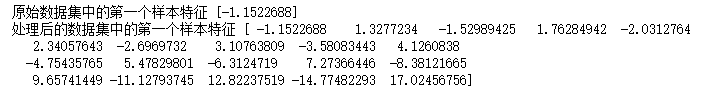【结果分析】

原始数据集的样本只有一个特征，而处理后的数据集有20个特征

验证一下结论：

#打印多项式特征的处理方式
print('PolynomialFeatures对原始数据的处理：',poly.get_feature_names())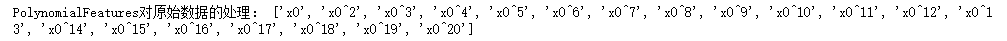#导入线性回归
from sklearn.linear_model import LinearRegression
#使用处理后的数据训练线性回归模型
LNR_poly = LinearRegression().fit(X_poly,y)
line_poly = poly.transform(line)
#绘制图形
plt.plot(line,LNR_poly.predict(line_poly),label='Linear Regressor')
plt.xlim(np.min(X)-0.5,np.max(X)+0.5)
plt.ylim(np.min(y)-0.5,np.max(y)+0.5)
plt.plot(X,y,'o',c='r')
plt.legend(loc='lower right')
plt.show()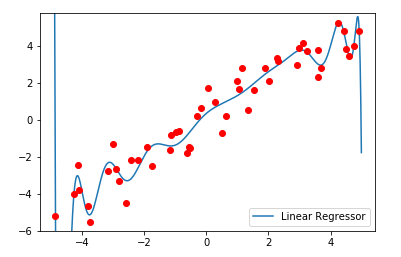【结果分析】

对于低维数据集，线性模型常常会出现欠拟合，将数据集进行多项式特征扩展后，可以一定程度上解决线性模型欠拟合的问题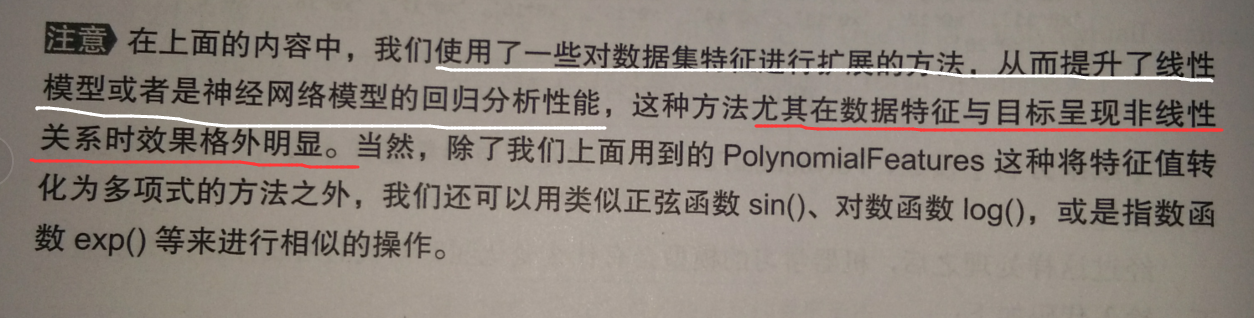1.使用单一变量法进行特征选择

【只适用于样本特征之间没有明显关联的情况】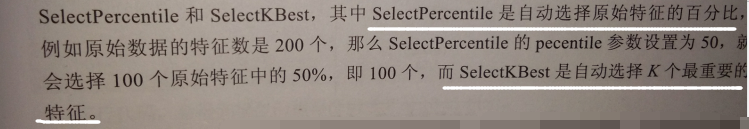用A股股票的交易数据集训练机器学习模型：

import pandas as pd
#读取股票数据集
#结果
print(stock.head())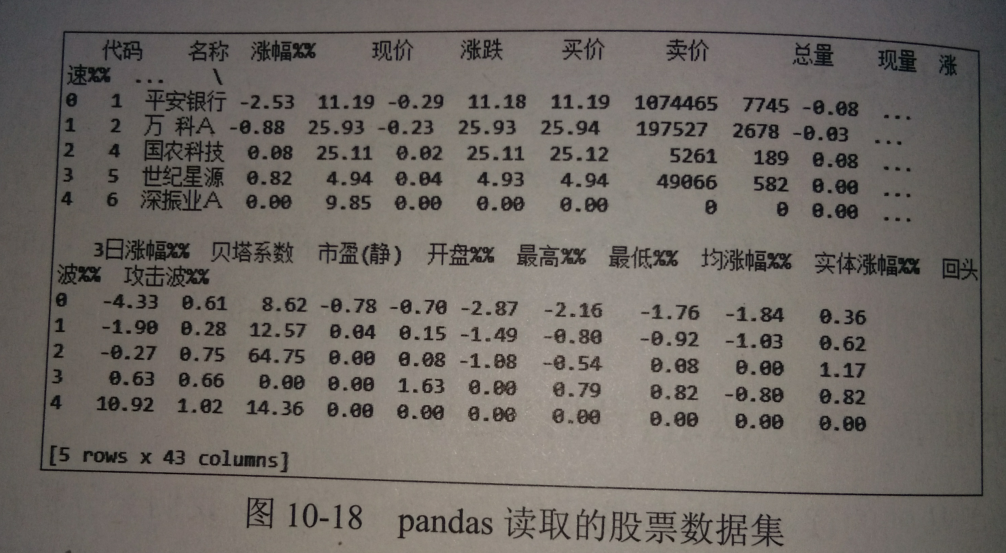【结果分析】

这个csv文件包括43列

#设置回归分析的目标为涨幅
y = stock['涨幅%%']
print(y.shape)
print(y)

（3421,）

-2.53

【结果分析】

一共有3421个样本，第一个样本，即“平安银行”的股票当日涨幅为-2.53%

#提取特征值
features = stock.loc[:,'现价':'流通股（亿）']
X = features.values
print(X.shape)
print(X[:1])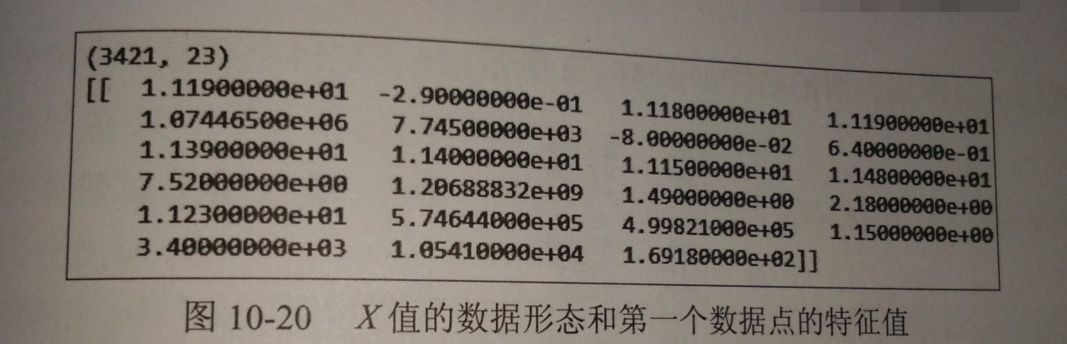【结果分析】

样本特征一共有23个，下面的数组是第一个样本的全部特征值

#导入数据集拆分工具
from sklearn.model_selection import train_test_spilt
#导入StandardScaler
from sklearn.preprocessing import StandardScaler
#设置神经网络隐藏层参数和alpha参数
mlpr=MLPRegreesor(random_state=62,hidden_layer_sizes=(100,100),alpha=0.001)
X_train,X_test,y_train,y_test = train_test_spilt(X,y,random_state=62)
#对数据进预处理
scaler = StandardScaler()
scaler.fit(X_train)
X_trian_scaled = scaler.transform(X_train)
X_test_scaled = scaler.transform(X_test)
#训练神经网络
mlpr.fit(X_train_scaled,y_train)
print(mlpr.score(X_test_scaled,y_test))

0.93

#列出涨幅>= 10%的股票
wanted = stock.loc[:,'名称']
print(wanted[y>=10])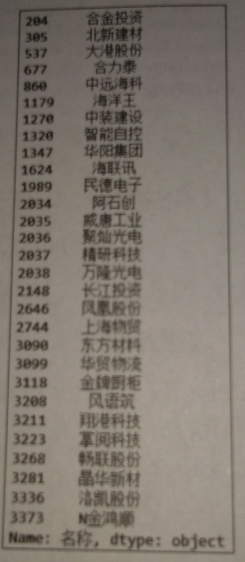#导入特征选择工具
from sklearn.features_selection import SelectPercentile
#设置特征选择参数
select = SelectPercentile(percenttile=50)
select.fit(X_train_scaled,y_train)
X_train_selected = select.transform(X_trian_scaled)
print('经过缩放的特征形态：',X_train_scaled.shape)
print('特征选择后的特征形态:',X_train_selected.shape)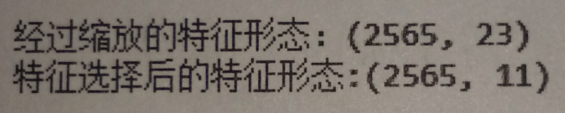#查看那些特征被保留下来
print(mask)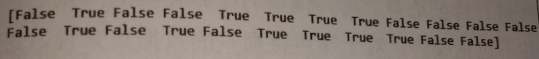【结果分析】

False表示该特征没有被选择

#使用图像表示特征选择的结果
plt.xlabel('Features Selected')
plt.show()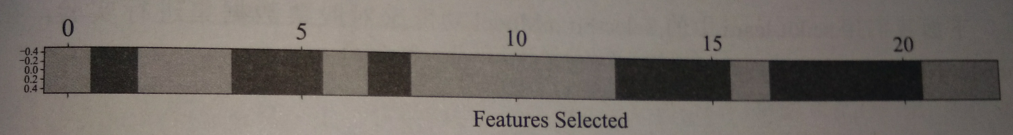#使用特征选择后的数据集训练神经网络
X_test_selected = select.transform(X_test_scaled)
mlpr_sp = MLPRegreesor(random_state=62,hidden_layer_sizes=(100,100),alpha=0.001)
mlpr_sp.fit(X_train_selected,y_train)
print('得分：',mlpr_sp.score(X_test_selected,y_test))

0.88

【结果分析】

进行特征选择后，模型的评分降低很正常，因为数据集并不包括噪声

【无论使用哪一个模型，“单一变量法”对数据进行处理的方式都是一样的，不依赖于使用什么算法进行建模】

2.基于模型的特征选择

原理————先使用一个有监督学习的模型对数据特征的重要性进行判断，然后把最重要的特征进行保留

【先用的进行判断重要性的模型和最终进行预测分析的模型不一定同一个】

#导入基于模型的特征选择工具
from sklearn.feature_selection import SelectFromModel
#导入随机森林模型
from sklearn.ensemble import RandomForestRegressor
#设置模型n_estimators参数
sfm = SelectionFormModel(RandomForestRegressor(n_estimators=100,random_state=38),threshold='median')
#使用模型拟合数据
sfm.fit(X_train_scaled,y_train)
X_train_sfm = sfm.transform(X_train_scaled)
print('基于随机森林模型进行特征后的数据形态',X_train_sfm.shape)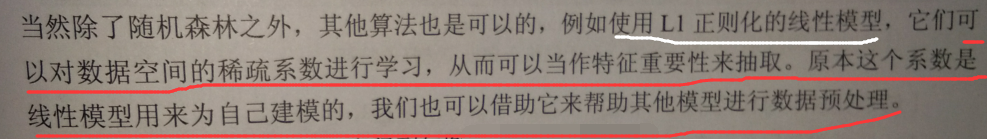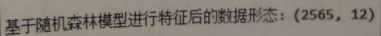【结果分析】

特征选择之后，数据集中样本的特征还剩下12个，少了11个

#显示保留的特征
print(mask_sfm)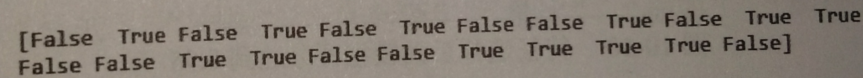【结果分析】

该特征选择保留了第2、4、6、9、11、12、15、16、19、20、21、22个特征

#对特征选择进行可视化
plt.xlabel('Features Selected')
plt.show()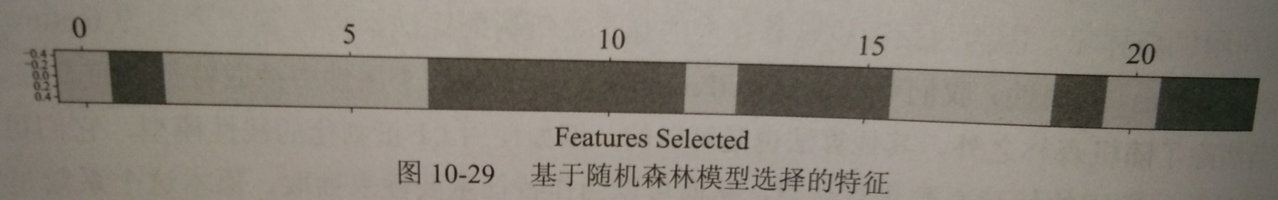【结果分析】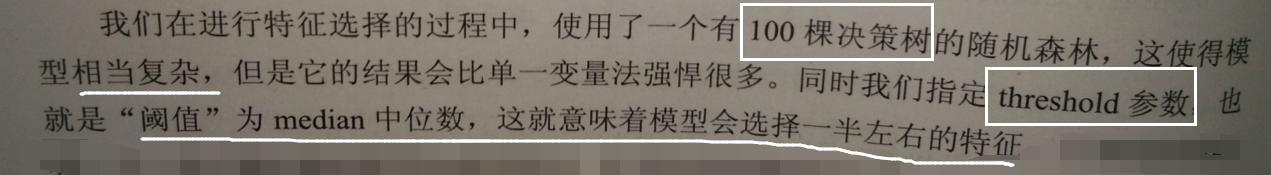#基于特征选择后的数据集训练神经网络
X_test_sfm = sfm.transform(X_test_scaled)
mlpr_sfm = MLPRegreesor(random_state=62,hidden_layer_sizes=(100,100),alpha=0.001)
mlpr_sfm.fit(X_train_sfm,y_train)
print('随机森林进行特征选择后的模型得分：',mlpr_sfm.score(X_test_sfm,y_test))

0.95

【结果分析】

在其他参数不变的情况下，得分比使用单一变量法进行特征选择的分数高很多

• 最开始，RFE对某个模型进行特征选择
• 之后再建立两个模型，其中一个对已经被选择的特征进行筛选
• 另一个对被剔除的模型进行筛选
• 一直重复，直到达到指定的特征数量

#导入RFE工具
from sklearn.feature_selection import RFE
rfe = RFE(RandomForestRegressor(n_estimators=100,random_state=38),n_features_to_select=12)
#使用RFE工具拟合数据
rfe.fit(X_train_scaled,y_trian)
#显示保留的特征
print(mask)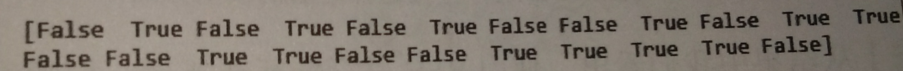#绘制RFE保留的特征
plt.xlabel('Features Selected')
plt.show()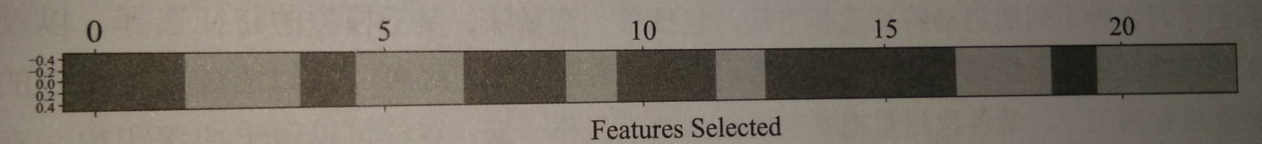【结果分析】

RFE选择的特征和单一变量法及基于单个模型的特征选择的结果都不相同

#使用新的数据集训练神经网络
X_trian_rfe = rfe.transform(X_train_scaled)
X_test_rfe = rfe.transform(X_test_scaled)
mlpr_rfe = MLPRegreesor(random_state62,hidden_layer_sizes=(100,100),alpha=0.001)
mlpr_rfe.fit(X_trian,y_trian)
print('RFE选择特征后的模型得分：',mlpr_rfe.score(X_test_rfe,y_test))

0.95

【结果分析】

不同的方法没有绝对的好与不好，只是适用不同场景

posted @ 2019-05-07 18:01  远征i  阅读(1007)  评论(0编辑  收藏